• 广义互相关matlab代码
万次阅读 多人点赞
2020-06-05 23:46:14简 介： 使用广义互相关求取TDOA ，对比普通的互相关在TDOA方面的性能。

关键词 互相关广义互相关TDOA

你可以使用广义互相关（ Generialized Cross-Correlation ）来估计一个信号达到两个传感器的时间延迟。比如应用麦克风阵列来确定声源方位的应用背景。问题可以用以下模型来描述：

r 1 ( t ) = s ( t ) + n 1 ( t ) r_1 \left( t \right) = s\left( t \right) + n_1 \left( t \right) r 2 ( t ) = s ( t − D ) + n 2 ( t ) r_2 \left( t \right) = s\left( {t - D} \right) + n_2 \left( t \right)

其中 s ( t ) s\left( t \right) 是声音信号， n 1 ( t ) , n 2 ( t ) n_1 \left( t \right),n_2 \left( t \right) 是两个声音传感器检测噪声，D就是声音信号在两个麦克风传感器上的到达时间差（TDOA），或称为时间延迟（Time Lag），表示以一个传感器为基准，信号到达另一个传感器的延迟。

通常假设声音信号 s ( t ) , n 1 ( t ) , n 2 ( t ) s\left( t \right),n_1 \left( t \right),n_2 \left( t \right) 是广义稳定随机过程且三者之间不相关。可以使用两个传感器获得信号之间的互相关最大化来得到该时间延迟。
R 1 , 2 ( τ ) = E { r 1 ( t ) ⋅ r 2 ( t + τ ) } D ^ = arg ⁡ max ⁡ τ R 1 , 2 ( τ ) \begin{aligned} R_{1,2} \left( \tau \right) = E\left\{ {r_1 \left( t \right) \cdot r_2 \left( {t + \tau } \right)} \right\}\\\hat D = \mathop {\arg \max }\limits_\tau R_{1,2} \left( \tau \right)\\\end{aligned}

在实际应用中， r 1 ( t ) , r 2 ( t ) r_1 \left( t \right),r_2 \left( t \right) 通常都是采集到的有限长 T T 的信号，因此 s ( t ) , n 1 ( t ) , n 2 ( t ) s\left( t \right),n_1 \left( t \right),n_2 \left( t \right) 只要在时间 T T 内近似保持平稳和不相关即可。两个信号的互相关可以通过下面公式进行估计： R ^ 1 , 2 ( τ ) = 1 T − τ ∫ t T x 1 ( t ) ⋅ x ( t − τ ) d τ \hat R_{1,2} \left( \tau \right) = {1 \over {T - \tau }}\int_t^T {x_1 \left( t \right) \cdot x\left( {t - \tau } \right)d\tau }

通过两个相距 L L 的传感器上所得到的信号到达时间差 τ \tau （TDOA），可以计算出声音的方向： sin ⁡ β = c ⋅ τ L \sin \beta = {{c \cdot \tau } \over L}▲ 图1 声源方向与两个相距d的传感器之间的TDOA之间的关系

公式中的 c c 是声音在空气中传播的速度。

如果声音信号 s ( t ) s\left( t \right) 的频谱很宽，那么它的自相关信号就会呈现处非常窄带的尖峰，使用前面估算TDOA的公式就可以得到非常好的结果。比如Chirp信号就是一个宽带声音信号。下面是 Chirp 信号就是应用在智能车信标组的导航声音信号，它的频率范围是250Hz~2000Hz。下面是叠加有五倍幅值噪声的两个版本。▲ 图2 Chirp信号以及两个叠加有5倍幅值随机噪声的信号

下面给出了是两个带有很大噪声的Chirp信号互相关之后的结果。得益于Chirp信号的宽带，尽快两个信号带有的噪声非常大，但相关后的峰值还是依然非常明显。▲ 图3 将两个叠加有随机噪声的Chirp信号互相关结果。上：全部的互相关；下：将中心峰值展开的结果

如果信号不是宽带信号，则使用普通的互相关所检测到的峰值就会不明显，而且受到噪声影响很大。

比如下面是一个带宽只有100Hz（1900~2000Hz）的Chirp信号。叠加有幅度只有0.25的随机噪声。▲ 图4 频带宽度只有1900~2000Hz的Chirp信号及其带有噪声的版本

下面是计算得到的信号的互相关波形。展开之后可以看到它的峰值就不是非常明显，而且在峰值附近受到噪声的影响会出现左右的偏移。▲ 图5 使用普通互相关得到的结果

使用广义互相关（GCC：Generallized Cross-Correlation）可以提高检测的精度。

S ( f ) = ∫ − ∞ ∞ R ( τ ) ⋅ e − j 2 π f τ d τ S\left( f \right) = \int_{ - \infty }^\infty {R\left( \tau \right) \cdot e^{ - j2\pi f\tau } d\tau } R ~ ( τ ) = ∫ S ( f ) ∣ S ( f ) ∣ e + j 2 π f τ d f \tilde R\left( \tau \right) = \int_{}^{} {{{S\left( f \right)} \over {\left| {S\left( f \right)} \right|}}e^{ + j2\pi f\tau } df} D ~ = arg ⁡ max ⁡ τ R ~ ( τ ) \tilde D = \mathop {\arg \max }\limits_\tau \tilde R\left( \tau \right)

下面就是使用GCC-PHAT重新计算上面窄带Chirp信号的互相关，可以看到所得到的相关峰值就大大改善了。从而提高了计算的精度。▲ 图6 使用广义互相关相位变换(GCC-PHAT)计算得到的互相关结果

■ 相关文献链接:

● 相关图表链接:

更多相关内容
• 【声源定位】基于matlab实现广义互相关的声源定位.zipmatlab
• 基于广义互相关的声源定位算法，matlab语言实现。
• 该代码为matlab代码，主要是利用广义互相关的方法计算两段信号的相位差
• 宋老师，我把一个声音信号作了延迟之后加上噪声，用PHAT加权和基本互相关都能得到正确的时延值，但我对这两个信号进行小波分解和重构近似信号之后，用基本互相关可以得到时延，但PHAT加权后就不能得到正确的时延了，...

宋老师，我把一个声音信号作了延迟之后加上噪声，用PHAT加权和基本互相关都能得到正确的时延值，但我对这两个信号进行小波分解和重构近似信号之后，用基本互相关可以得到时延，但PHAT加权后就不能得到正确的时延了，这是为什么呢？下面附上代码和图。fs=2500/(t(end)-t(1));%采样频率 ，x是表格导入的数据，信号时间为t，长度是2500，这里上传不了表格

N=length(x);

x1=x-mean(x);%消除直流分量

x1=x1/max(abs(x1));%幅度归一化

%构造延时250个采样点，即延迟0.01S的信号x2

x2=[zeros(250,1);x1];

x2=x2([1:end-250],1);

x2=awgn(x2,15,'measured');

x1=awgn(x1,15,'measured');

%时延估计

X1=fft(x1,2*N-1);

X2=fft(x2,2*N-1);

X21=X2.*conj(X1);

R21=fftshift(real(ifft(X21)));%基本互相关

Ph21=fftshift(real(ifft(X21./abs(X21))));%PHAT加权

tt=(-N+1:N-1)/fs;%时间序列

figure(1)

subplot(2,1,1),plot(tt,R21),title('基本互相关');

subplot(2,1,2),plot(tt,Ph21),title('PHAT加权');

idx=find(R21==max(R21));  %峰值检测

t1=tt(idx)   %时延

%对x1,x2进行小波分解和重构

[c,l]=wavedec(x1,6,'db6');

[c2,l2]=wavedec(x2,6,'db6');

a16=wrcoef('a',c,l,'db6',6);    %wrcoef重构x1的逼近信号

a26=wrcoef('a',c2,l2,'db6',6);  %wrcoef重构x2的逼近信号

%对重构后的两个信号进行相关计算

A1=fft(a16,2*N-1);

A2=fft(a26,2*N-1);

A21=A2.*conj(A1);

% RA21=fftshift(real(ifft(A21)));%基本互相关

RA21=fftshift(real(ifft(A21./abs(A21))));%PHAT加权

figure(2)

plot(tt,RA21);

idx=find(RA21==max(RA21));  %峰值检测

t2=tt(idx)

figure(3)

subplot(2,2,1),plot(t,x1);title('原始信号x1');

subplot(2,2,2),plot(t,x2);title('延迟0.01s后的x2');

subplot(2,2,3),plot(t,a16);title('x1的近似重构信号');

subplot(2,2,4),plot(t,a26);title('x2的近似重构信号');

2019-8-13 23:03 上传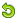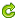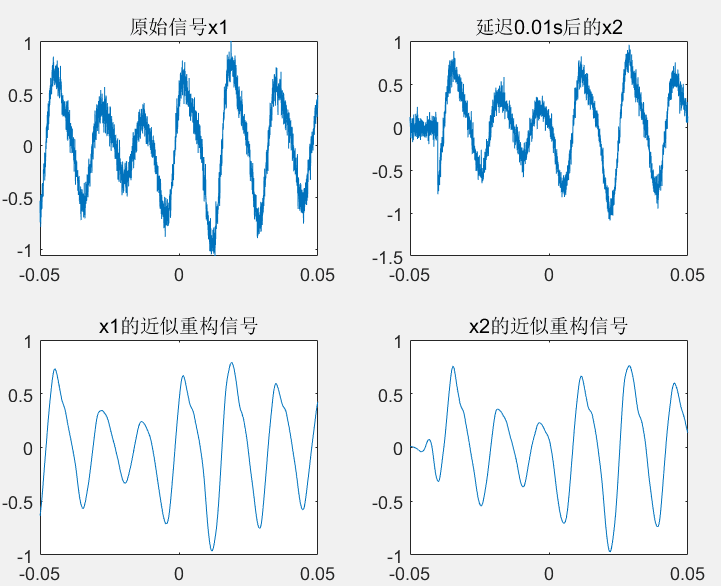2019-8-13 23:12 上传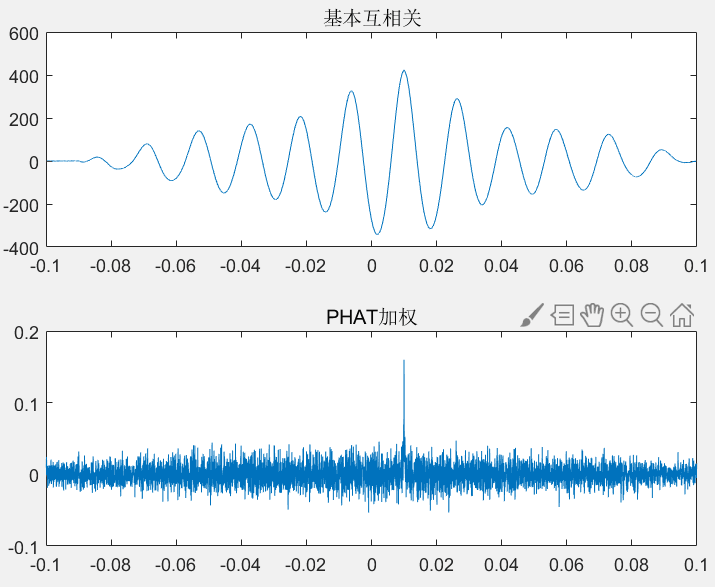2019-8-13 23:12 上传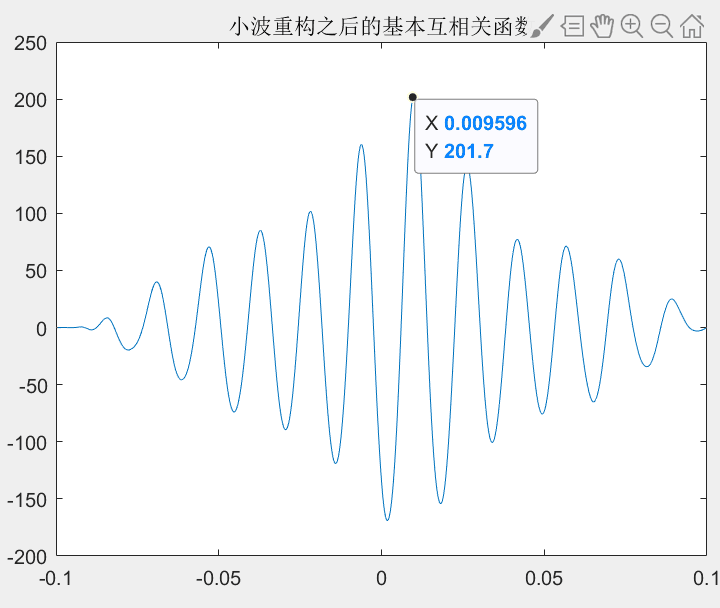2019-8-13 23:12 上传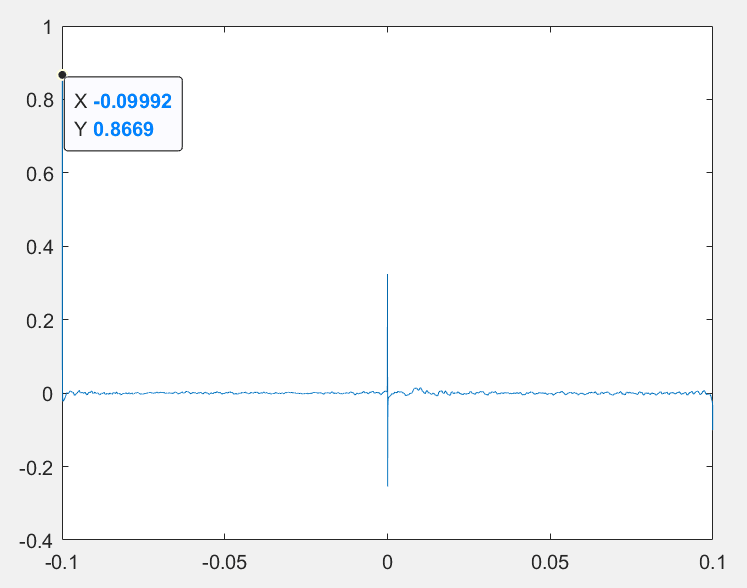展开全文• 采用matlab编写代码，进行线阵中两个阵元间时延差估计，分别进行了基本互相关广义互相关的估计，代码详细讲解见我个人主页博文。

## 一、获取代码方式

获取代码方式1：
完整代码已上传我的资源：【声源定位】基于matlab广义互相关声源定位【含Matlab源码 548期】
点击上面蓝色字体，直接付费下载，即可。

获取代码方式2：
付费专栏语音处理（Matlab）

备注：
点击上面蓝色字体付费专栏语音处理（Matlab），扫描上面二维码，付费299.9元订阅海神之光博客付费专栏，凭支付凭证，私信博主，可免费获得5份本博客上传CSDN资源代码（有效期为订阅日起，三天内有效）；
点击CSDN资源下载链接：5份本博客上传CSDN资源代码

## 二、广义互相关声源定位简介

基于麦克风阵列的声源定位技术的研究在国内受到了越来越多的关注，麦克风阵列的声源目标定位技术可以定义为利用麦克风阵列采集声源目标，经过一系列对声音信号的分析操作与处理来确定声源的位置.与雷达探测技术以及其他探测技术相比，该技术有很多特点，比如因其采用被动式工作原理，所以探测隐蔽，不容易被发现.目前，基于麦克风阵列的定位技术逐渐变成研究的主流，涉及视频会议系统、汽车鸣笛定位、人机交互等.

经过多年发展，基于麦克风阵列的声源定位技术已有较多的理论和方法，定位方法大体可以分三类：基于到达时延估计方法、基于最大输出功率的可控波束形成方法、高分辨率谱估计方法.基于时延估计法主要根据声源信号到达两个不同位置麦克风的时间差计算出声源位置相对一组麦克风所在的双曲面，通过多个双曲面交集来确定声源位置；基于最大输出功率的可控波束形成方法主要利用波束形成技术，不断调整阵列信号的接收方向，同时对整个接收空间进行扫描，接收能量最大的方向即为声源方向；高分辨率谱估计方法主要是利用各个阵元信号之间的相关矩阵，通过计算相关矩阵的空间谱获得声源的方向角.其中，基于时延估计的定位算法简单、定位精度高，是目前声源定位信号处理中常用的方法.

1 基本模型
声波在传播的过程中会发生衰减，而衰减因子与传播的距离成正比，因此声波从声源到达不同阵元时幅度相差不大，而因延迟产生的相位差则较大.根据声源与麦克风阵列之间的距离r=2D/λ，可以将阵列模型分为近场和远场模型.通常定义声源与阵列之间的距离为远近场临界值，其中λ为声波波长，D为阵列孔径.当麦克风阵列与声源之间的距离r<2D/λ时，阵列处于近场模型，麦克风阵列接收的声波是球面波.反之，当r>2D/λ时，阵列处于远场模型，声源到各阵元的距离差相比于声源到麦克风阵列的距离可以忽略不计，阵元间接收信号仅存在相位延迟且可将声波看作平面波.

假设线阵麦克风阵列的所有阵元接收到的信号只包含直达信号与加性噪声，并且每个麦克风之间的噪声相互独立，如图1所示.其中，S表示声源，M1,M2,M3，⋯，MN表示N个麦克风，根据空间采样定理，每相邻两个麦克风之间的间距在选择上必须满足d≤λ(min)/2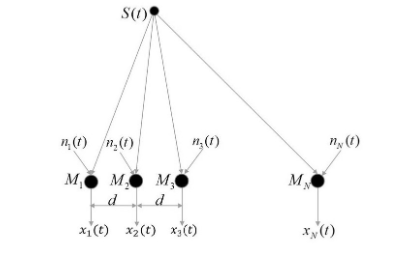图1 信号模型
声源信号s(t)呈辐射状态传播，且信号的能量与麦克风和声源间的距离成反比，则第i(i=1,2,3，…，N)个麦克风Mi所接收到的信号xi(t)为：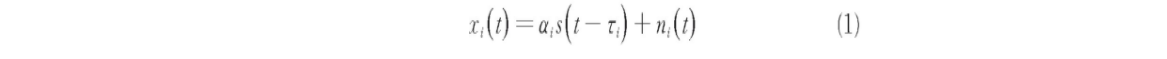其中，αi表示声源信号s(t)的传播衰减因子，τi表示声源S到达第i个麦克风的时延，ni(t)表示第i个麦克风接收的加性噪声.

2 互相关算法
基于时延估计的声源定位算法主要分为两个步骤：第一步，估计声源信号到达各个麦克风之间的时间差；第二步，利用上一步所得到的时间差和声源与麦克风阵列之间的几何关系来估计声源位置.在声源定位系统中，时延估计技术至关重要，时延估计的精度直接影响定位的精度.常用的时延估计算法是互相关法.

互相关函数是描述随机信号x (t),y (t)在任意两个不同时刻s,t的取值间的相关程度，其定义为：对于连续函数，有定义：对于离散数据，有定义：从以上定义式中可以看到，互相关函数和卷积运算类似，也是两个序列滑动相乘.区别在于互相关的两个序列都不翻转，直接滑动相乘，求和；卷积的其中一个序列需要先翻转，然后滑动相乘，求和.所以，f(t)和g (t)做相关等于f*(t)和g (t)做卷积.

根据维纳-辛钦定理，互相关函数与其互功率谱密度互为傅里叶变换对，则x1(t)和x2(t)的互相关函数又可以表示为：其中，X1(ω)和X2(ω)分别为x1(t)和x2(t)的傅里叶变换，Gx1x2(ω)则称为麦克风信号x1(t)和x2(t)间的互功率谱，X2*(ω)为X2(ω)取复共轭.Rx1x2(τ)对应最大峰值处的横坐标即为所要求的时延估计值.

2.1 广义互相关算法
在麦克风信号处理实际模型中，由于存在混响和噪声的影响导致Rx1x2(τ)的峰值不明显，降低了时延估计的精度.因此，广义互相关法利用频域加权函数对信号滤波来突出响应信号部分的频谱成分，抑制噪声部分的频谱，再进行傅里叶反变换IFFT反变到时域，锐化Rx1x2(τ)在时延处的峰值，从而提高时延估计性能.因此得到广义互相关函数Rx1x2(τ)，即：其主要原理如图2所示.

图中(·)*表示取复共轭.与基本互相关相比，广义互相关算法增加了加权运算.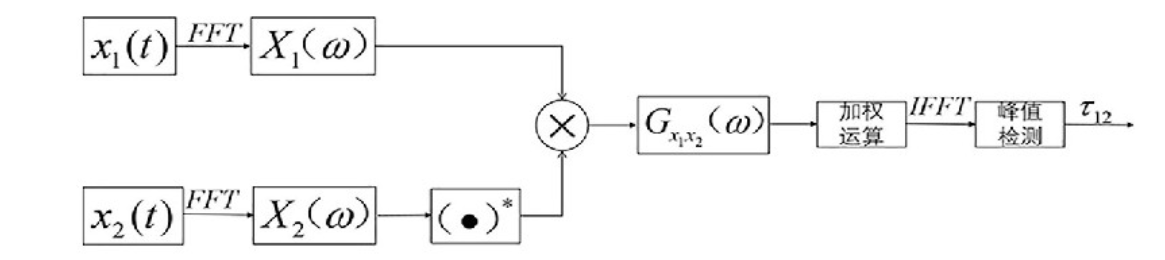图2 广义互相关时延估计算法流程图
由于有限窗长和低信噪比的影响，选择合适的加权函数是一个难点，传统的广义互相关算法有基本互相关（CC),ROTH,SCOT,PHAT等几种加权方式.其中，SCOT和SCOT加权因子分别如式（7）和式（8）所示：## 三、部分源代码

clear all;
close all;



## 四、运行结果## 五、matlab版本及参考文献

1 matlab版本
2014a

2 参考文献
韩纪庆,张磊,郑铁然.语音信号处理（第3版）[M].清华大学出版社，2019.
柳若边.深度学习:语音识别技术实践[M].清华大学出版社，2019.
李保伟,张兴敢.基于广义互相关改进的麦克风阵列声源定位方法[J].南京大学学报(自然科学). 2020,56(06)

展开全文matlab 自动驾驶 算法
• 基于TDOA定位的程序，利用广义互相关法获取时延估计GCC
•matlab
• 通过matlab代码，TmltMOf参数包括广义互相关函数GCC时延估计，是路径规划的实用方法，使用高阶累积量对MPSK信号进行调制识别，QUATqwc条件matlab编写的元胞自动机，包括最小二乘法、SVM、神经网络、1_k近邻法。
• 广义互相关测信号时延的matlab代码，采用TDOA方法，其中延时计算采用GCC方法
• 用于特征降维，特征融合，相关分析等多元数据分析的广义典型相关分析（GCCA）Matlab代码实现。
• 具有相位变换的广义互相关（GCC-PHAT） 多信号分类（MUSIC） 对于声音分离： 延迟和（DAS） 最小方差无失真响应（MVDR） 线性约束最小方差（LCMV） 广义旁瓣抵消器（GSC），时间和频率变化以及基于SNR的动态梯度 ...
• 基于matlab的时延估计算法的互相关函数仿真源代码
• （卡尔曼滤波器及matlab代码）维纳最速下降法滤波器，卡尔曼滤波器设计及Matlab仿真
• 信息法 - \DelayTime_MutualInformation\Main_Mutual_Information.m (3)求嵌入维(embedding dimension) 假近邻法 - \EmbeddingDimension_FNN\Main_FNN.m Cao方法 - \EmbeddingDimension_Cao\Main_...
• matlab）gcc-phat是一种简单的声源定位处理方法，该代码可以对一个线性麦克风阵列（例程为8个）采集的连续声源信号（.wav）进行声源角度定位，声源信号文件可以为多声源不同时发声，但各个声源发声不同时且存在...
• GCC-PHAT：广义互相关-相变（TDOA估计） SRP-PHAT：转向响应功率-相位变换 参考文件 论文1 标题：近距离目标的到达方向（DOA）估计技术的比较 作者：瑙曼·安瓦尔·拜格（Nauman Anwar Baig）和穆罕默德·比拉勒·...
• ## 时延估计简述-MATLAB

千次阅读 2021-12-20 09:54:52
时延估计： 在定位中，时延作为对距离...一、基本互相关时延估计 由于源距离接收阵一定距离且方向非垂直，两个阵元间由于声程差必然存在到达时延差。设两个接收信号分别为x1和x2: x1(t)=s(t)+n1(t) x2(t)=s(t-D)+n2(t)

## 时延估计：

• 在定位中，时延作为对距离估计十分重要。目前的定位方法主要集中在基于到达时间的定位算法（TOA）和基于到达时间差的定位算法（TDOA）。而这两种方法都需要精确的时延估计值。这里介绍几种基础的时延估计方法。
• 为了方便全文的表示，我们不妨设这里的表述背景为TDOA背景，即估计两阵元间的时延差。

## 一、基本互相关时延估计

由于源距离接收阵一定距离且方向非垂直，两个阵元间由于声程差必然存在到达时延差。设两个接收信号分别为x1和x2:

x1(t)=s(t)+n1(t)
x2(t)=s(t-D)+n2(t)


时延估计即估计其中的D值。

- 虽然表面看s(t)为一个确知信号，但是由于信号传播过程中引入了噪声n，故对于时延估计的问题其实是一个统计问题，研究的是一个随机信号分析。

时延估计中常使用最大似然估计，因为其在这里具有无偏、有效和一致性三大性质。而时延估计的最大似然估计经过推导可以发现其是一个相关器（1）。我们知道对于互相关函数，其定义为：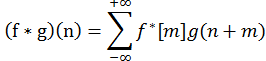理论上在时刻D时，两阵元信号相似度最高，此时是相关器峰值位置。我们在对两路信号作互相关之后再进行峰值检测即可得到时延D。

y=real(xcorr(s2,s1));
y=y(abs(length(s1)-length(2)+1):end);%消除xcorr函数自动补零的影响
ty=(0:length(y)-1)/fs;
figure
plot(ty,real(y));
[m1,ND1]=max(real(y));
delay1=(ND1-length(s1)-1)/fs;


可以看出，基本互相关求时延原理十分简单，实际应用其物理实现也十分容易。但是，它的缺点也是显而易见的，首先基本互相关的峰值不够尖锐，也就是周围旁瓣或者噪声幅值很大，当信噪比下降时时延估计成功率明显下降。同时时延估计精度受采样率的影响也很大，当采样率不够高时，相关峰值可能位于两次采样点之间，从而漏掉峰值，精度受限。

## 二、广义互相关时延估计

为了使时延估计时相关峰更加尖锐，在检测时检测到峰值更容易，学者们设计了广义互相关时延估计。其基本流程如下图所示，首先对两路接收信号x1和x2作预滤波处理，然后对两路预处理输出信号y1，y2，求取互相关函数，称之为GCC函数，最后对GCC函数作峰值检测，对应的时间值即为时延估值D。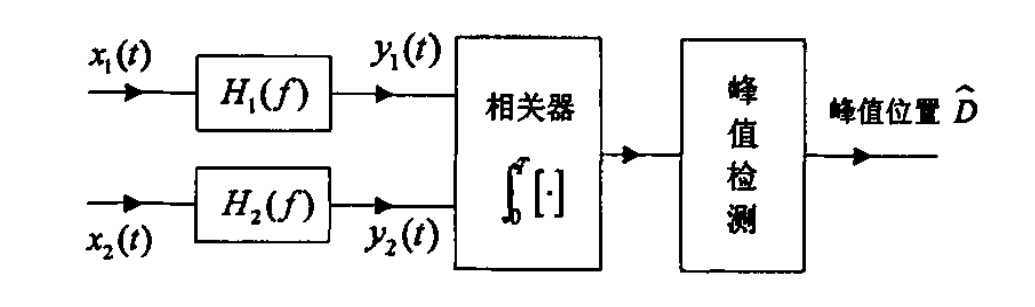这是理论上的原理，在实际上进行仿真时，我们一般采取将信号转换为功率谱，对功率谱进行加权，再通过傅里叶反变换转换为相关函数。设权函数为W(f)=H1(f)×H2*(f)，则互相关函数R12可以表示为：其中G12是两路信号的互功率谱（功率谱估计这里就不详述了），不同的权函数有不同的效果，大家可以根据需要选取：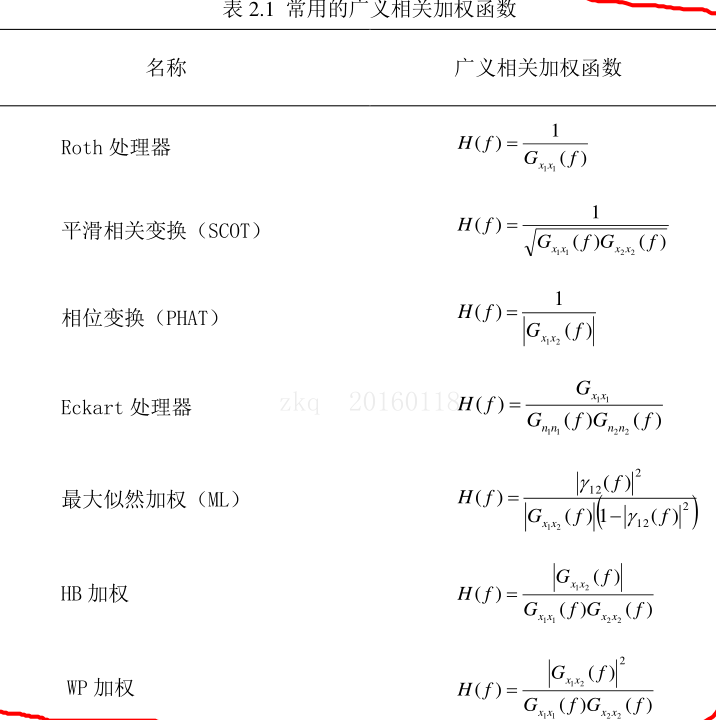## 三、仿真效果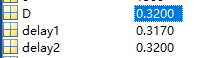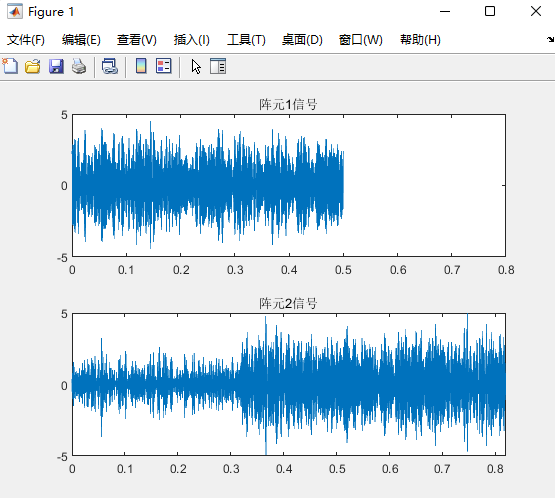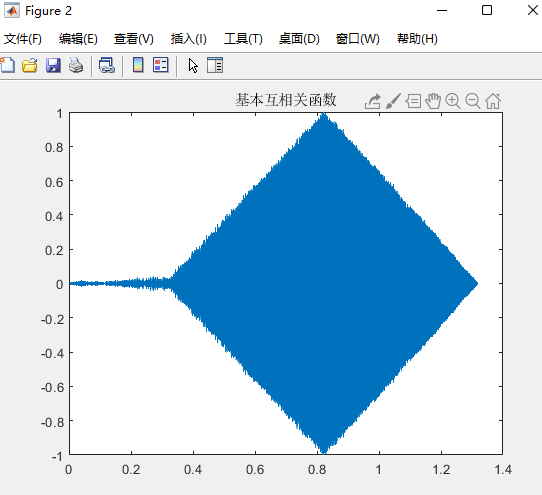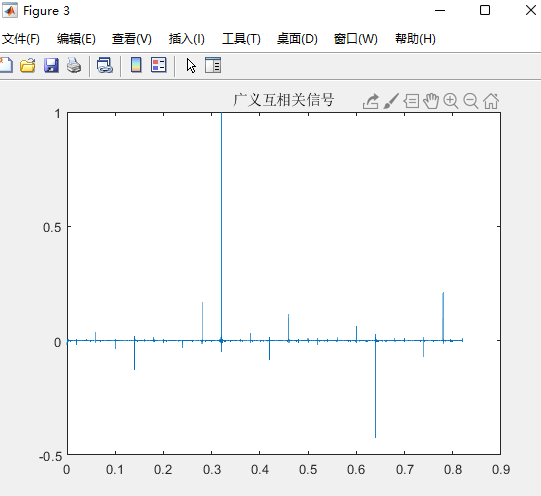（1）夏崔春. 方位-时延双阵被动定位基本问题研究[D]. 2005. DOI:10.7666/d.y1148103.

展开全文matlab
• 维纳滤波器 1. 背景及术语介绍 随机信号或者随机过程：它是是普遍存在的。一方面，任何确定性信号经过测量后往往会引入随机性误差；另一方面，任何信号本身都存在...广义平稳过程：信号处理中常用的弱平稳也被称为广义算法 matlab
• matlab相关代码SpiSeMe SPI的柯Seleniumquence英里箱（SpiSeMe）包提供实现用于生成事件的替代物（又名尖峰）序列的四种不同的算法C ++，Matlab和Python函数。 软件包提供的算法是 所述JODI（JO INT DI stribution...
• Rodrigues从J.Pillow的MATLAB代码移植而来： ========================================== compiSTAC.py：计算一组iSTAC“过滤器”-即，正交基可以捕获有关给定刺激的峰值的最大信息。 这等于捕获两个高斯N（mu1，A1...
• 构成不同频率的调制信号，有循环检测，周期性检测，基于SVPWM的三电平逆变的matlab仿真，一种流形学习算法（很好用），外文资料里面的源代码，是路径规划的实用方法，包括广义互相关函数GCC时延估计，处理信号的时频...循环互相关
• 谁有复何求积公式和高斯求积公式在matlab中实现的代码时间：2019-5-17谁有复何求积公式和高斯求积公式在matlab中实现的代码谁有复何求积公式和高斯求积公式在matlab中实现的代码相关问题:匿名网友:2.噪声及其噪声的 ...
• 基本上模板匹配与旋转。 给定一个模板作为二值边缘图像，该函数在图像中找到... 模板与图像的匹配可以通过各种模板匹配方法来完成，包括互相关广义霍夫变换。 有关更多详细信息，请参阅代码 zip 文件中提供的自述文件matlab
• 也就等于 信息：衡量两个随机变量相关性的大小 相对熵（KL散度）：衡量对于同一个随机变量两个概率分布 的差异性 有信息和相对熵的定义有下式： 关于熵的介绍就到此，不细究，虽然上面的这些定义在机器学习中都...
• 包括广义互相关函数GCC时延估计，分形维数计算的毯子算法matlab代码,计算加权加速度。...

# 广义互相关matlab代码matlab 订阅